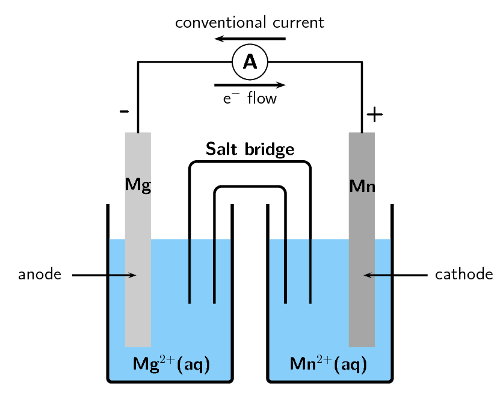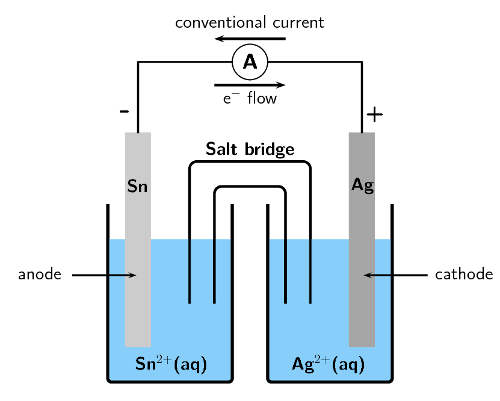Home Practice
For learners and parents For teachers and schools
Textbooks
Full catalogue
Pricing SupportLog in

We think you are located in United States. Is this correct?

# 13.4 Processes in electrochemical cells

## 13.4 Processes in electrochemical cells (ESCR7)

### Half-cells and half-reactions (ESCR8)

Galvanic cells are actually made up of two half-cells. One half-cell contains the anode and an electrolyte containing the same metal cations. The other half-cell contains the cathode and an electrolyte containing the same metal cations. These half-cells are connected by a salt-bridge and the electrodes are connected through an external circuit.

Earlier in this chapter we discussed a zinc-copper cell. This was made up of a zinc half-cell, containing a zinc electrode and a zinc(II) sulphate ($$\text{ZnSO}_{4}$$) electrolyte solution, and a copper half-cell, containing a copper electrode and a copper(II) sulphate solution.

Half-cell

A half-cell is a structure that consists of a conductive electrode surrounded by a conductive electrolyte.

In each half-cell a half-reaction takes place:

• Copper plate

At the copper plate, there was an increase in mass. This means that $$\text{Cu}^{2+}$$ ions from the copper(II) sulfate solution were deposited onto the plate as atoms of copper metal. The half-reaction that takes place at the copper plate is:

$$\text{Cu}^{2+}(\text{aq}) + 2\text{e}^{-}$$ $$\to$$ $$\text{Cu}(\text{s})$$

As electrons are gained by the copper ions this is the $$\color{red}{\textbf{reduction half-reaction}}$$.

• Zinc plate

At the zinc plate, there was a decrease in mass. This means that some of the solid zinc goes into solution as $$\text{Zn}^{2+}$$ ions. The electrons remain on the zinc plate, giving it a negative charge. The half-reaction that takes place at the zinc plate is:

$$\text{Zn}(\text{s})$$ $$\to$$ $$\text{Zn}^{2+}(\text{aq}) + 2\text{e}^{-}$$

As electrons are lost by the zinc atoms this is the $$\color{blue}{\textbf{oxidation half-reaction}}$$.

• The overall reaction

You can then combine the two half-reactions from these two half-cells to get the overall reaction:

$$\color{blue}{\text{Zn(s)}} + \color{red}{\text{Cu}^{2+}\text{(aq)}} + \color{red}{\text{2e}^{-}} \to \color{blue}{\text{Zn}^{2+}\text{(aq)}} + \color{red}{\text{Cu(s)}} + \color{blue}{\text{2e}^{-}}$$ or, if we cancel the electrons:

$$\color{blue}{\text{Zn(s)}} + \color{red}{\text{Cu}^{2+}\text{(aq)}} \to \color{blue}{\text{Zn}^{2+}\text{(aq)}} + \color{red}{\text{Cu(s)}}$$

It is possible to look at the half-reaction taking place in a half-cell and determine which electrode is the anode and which is the cathode.

• $$\color{blue}{\textbf{Oxidation}}$$ is loss at the $$\color{blue}{\textbf{anode}}$$, therefore the oxidation half-reaction occurs in the half-cell containing the anode.

• $$\color{red}{\textbf{Reduction}}$$ is gain at the $$\color{red}{\textbf{cathode}}$$ so the reduction half-reaction occurs in the half-cell containing the cathode.

Remember that for an electrochemical cell the standard cell notation is:

$$\color{blue}{\textbf{Zn(s)}}$$$$|$$$$\color{blue}{\textbf{Zn}^{2+}\textbf{(aq)}}$$$$||$$$$\color{red}{\textbf{Cu}^{2+}\textbf{(aq)}}$$$$|$$$$\color{red}{\textbf{Cu(s)}}$$

$$|$$ = a phase boundary (solid/aqueous)

$$||$$ = the salt bridge

## Worked example 4: Understanding galvanic cells

For the following cell:

$$\text{Zn}(\text{s})|\text{Zn}^{2+}(\text{aq})||\text{Ag}^{+}(\text{aq})|\text{Ag}(\text{s})$$

1. Give the anode and cathode half-reactions.

2. Write the overall equation for the chemical reaction.

3. Give the direction of the current in the external circuit.

### Identify the oxidation and reduction reactions

By convention in standard cell notation, the anode is written on the left and the cathode is written on the right. So, in this cell:

Zinc is the anode (solid zinc is oxidised).

Silver is the cathode (silver ions are reduced).

### Write the two half-reactions

Oxidation is loss of electrons at the anode: $$\text{Zn}(\text{s})$$ $$\to$$ $$\text{Zn}^{2+}(\text{aq}) + 2\text{e}^{-}$$

Reduction is gain of electrons at the cathode: $$\text{Ag}^{+}(\text{aq}) + \text{e}^{-}$$ $$\to$$ $$\text{Ag}(\text{s})$$

### Combine the half-reactions to get the overall equation

Balance the charge by multiplying the reduction half-reaction by $$\text{2}$$.

$$2\text{Ag}^{+}(\text{aq}) + 2\text{e}^{-}$$ $$\to$$ $$2\text{Ag}(\text{s})$$

$$\text{Zn}(\text{s}) + 2\text{Ag}^{+}(\text{aq})$$ $$\to$$ $$\text{Zn}^{2+}(\text{aq}) + \text{Ag}(\text{s})$$

### Determine the direction of current flow

The solid zinc is oxidised to form zinc ions. These electrons are left on the zinc electrode (anode) making it negative.

The silver ions take electrons and are reduced to form solid silver. This makes the silver electrode (cathode) positive.

Electron flow is from negative to positive, so from the anode to the cathode. Conventional current is in the opposite direction to electron flow. Therefore current will flow from the cathode (silver) to the anode (zinc).

temp text

## Galvanic cells

Textbook Exercise 13.5

The following half-reactions take place in an electrochemical cell:

$$\text{Fe}(\text{s})$$ $$\to$$ $$\text{Fe}^{3+}(\text{aq}) + 3\text{e}^{-}$$

$$\text{Fe}^{2+}(\text{aq}) + 2\text{e}^{-}$$ $$\to$$ $$\text{Fe}(\text{s})$$

Which is the oxidation half-reaction?

Oxidation is the loss of electrons.

$$\text{Fe}(\text{s})$$ $$\to$$ $$\text{Fe}^{3+}(\text{aq}) + 3\text{e}^{-}$$

Which is the reduction half-reaction?

Reduction is the gain of electrons.

$$\text{Fe}^{2+}(\text{aq}) + 2\text{e}^{-}$$ $$\to$$$$\text{Fe}(\text{s})$$

Name the oxidising agent.

An oxidising agent causes oxidation and is itself reduced.

$$\text{Fe}^{2+}(\text{aq})$$

Name the reducing agent.

A reducing agent causes reduction and is itself oxidised.

$$\text{Fe}(\text{s})$$

Use standard notation to represent this electrochemical cell.

Oxidation is loss at the anode, therefore $$\text{Fe}(\text{s})$$ in oxidation half-reaction is the anode.

Reduction is gain at the cathode, therefore $$\text{Fe}(\text{s})$$ in reduction half-reaction is the cathode.

$$\text{Fe}(\text{s})|\text{Fe}^{3+}(\text{aq})||\text{Fe}^{2+}(\text{aq})|\text{Fe}(\text{s})$$

For the following cell:

$$\text{Mg}(\text{s})|\text{Mg}^{2+}(\text{aq})||\text{Mn}^{2+}(\text{aq})|\text{Mn}(\text{s})$$

Give the cathode half-reaction.

The cathode is written on the right, therefore manganese is the cathode. Reduction is gain at the cathode.

$$\text{Mn}^{2+}(\text{aq}) + 2\text{e}^{-}$$ $$\to$$ $$\text{Mn}(\text{s})$$

Give the anode half-reaction.

The anode is written on the left, therefore magnesium is the anode. Oxidation is loss at the anode.

$$\text{Mg}(\text{s})$$ $$\to$$ $$\text{Mg}^{2+}(\text{aq}) + 2\text{e}^{-}$$

Give the overall equation for the electrochemical cell.

The charge of the two half-reactions is balanced.

$$\text{Mg}(\text{s}) + \text{Mn}^{2+}(\text{aq})$$ $$\to$$ $$\text{Mg}^{2+}(\text{aq}) + \text{Mn}(\text{s})$$

What metals could be used for the electrodes in this electrochemical cell?

Magnesium metal and manganese metal

Suggest two electrolytes for this electrochemical cell.

Magnesium sulfate and manganese sulfate, or magnesium nitrate and manganese nitrate

In which direction will the current flow?

The solid $$\text{Mg}$$ is oxidised to form $$\text{Mg}^{2+}$$ ions at the anode. This makes the anode negative.

The $$\text{Mn}^{2+}$$ ions are reduced to form $$\text{Mn}(\text{s})$$ at the cathode. This makes the cathode positive.

Electron flow is from negative to positive, so from the anode to the cathode. Conventional current is in the opposite direction (from cathode to anode).

Therefore conventional current is from the manganese plate to the magnesium plate.

Draw a simple sketch of the complete cell.For the following cell:

$$\text{Sn}(\text{s})|\text{Sn}^{2+}(\text{aq})||\text{Ag}^{+}(\text{aq})|\text{Ag}(\text{s})$$

Give the cathode half-reaction.

The cathode is written on the right, therefore silver is the cathode. Reduction is gain at the cathode.

$$\text{Ag}^{+}(\text{aq}) + \text{e}^{-}$$ $$\to$$ $$\text{Ag}(\text{s})$$

Give the anode half-reaction.

The anode is written on the left, therefore tin is the anode. Oxidation is loss at the anode.

$$\text{Sn}(\text{s})$$ $$\to$$ $$\text{Sn}^{2+}(\text{aq}) + 2\text{e}^{-}$$

Give the overall equation for the electrochemical cell.

To balance the charges the cathode half-reaction must be multiplied by $$\text{2}$$.

$$2\text{Ag}^{+}(\text{aq}) + 2\text{e}^{-}$$ $$\to$$ $$2\text{Ag}(\text{s})$$

$$\text{Sn}(\text{s}) + 2\text{Ag}^{+}(\text{aq})$$ $$\to$$ $$\text{Sn}^{2+}(\text{aq}) + 2\text{Ag}(\text{s})$$

Draw a simple sketch of the complete cell.## Worked example 5: Reactions at the anode and cathode

A cell contains a silver anode and a copper cathode. Give the half-cell reactions occurring at the anode and cathode, as well as standard cell notation for this cell.

### What type of reaction occurs at the anode, and what type occurs at the cathode?

Oxidation is loss of electrons at the anode. So the silver anode will be oxidised.

Reduction is gain of electrons at the cathode. So the copper cathode will be reduced.

### Write down the half-reactions as they would occur in the cell

$$\text{Ag}(\text{s})$$ $$\to$$ $$\text{Ag}^{+}(\text{aq}) + \text{e}^{-}$$ (oxidation half-reaction)

$$\text{Cu}^{2+}(\text{aq}) + 2\text{e}^{-}$$ $$\to$$ $$\text{Cu}(\text{s})$$ (reduction half-reaction)

### Give the standard cell notation for this reaction

The anode is always written first (on the left): $$\text{Ag}(\text{s})|\text{Ag}^{+}(\text{aq})$$

The cathode is always written second (on the right): $$\text{Cu}^{2+}(\text{aq})|\text{Cu}(\text{s})$$

Therefore the standard cell notation is:

$$\text{Ag}(\text{s})|\text{Ag}^{+}(\text{aq})||\text{Cu}^{2+}(\text{aq})|\text{Cu}(\text{s})$$

## Worked example 6: Determining overall reactions

The following half-reactions take place in a cell:

$$\text{Fe}(\text{s})$$ $$\to$$ $$\text{Fe}^{2+}(\text{aq}) + 2\text{e}^{-}$$

$$\text{Cu}^{2+}(\text{aq}) + 2\text{e}^{-}$$ $$\to$$ $$\text{Cu}(\text{s})$$

Determine the overall reaction that takes place as a balanced chemical equation and in standard cell notation.

### Identify the oxidation and reduction half-reactions

$$\text{Fe}(\text{s})$$ $$\to$$ $$\text{Fe}^{2+}(\text{aq}) + 2\text{e}^{-}$$ (oxidation half-reaction)

$$\text{Cu}^{2+}(\text{aq}) + 2\text{e}^{-}$$ $$\to$$ $$\text{Cu}(\text{s})$$ (reduction half-reaction)

### Which metal is the anode and which is the cathode?

Oxidation is loss of electrons at the anode, therefore $$\text{Fe}$$ is the anode. Reduction is gain of electrons at the cathode, therefore $$\text{Cu}$$ is the cathode.

### Compare the number of electrons in each equation

There are 2 electrons in both equations, so the charges are balanced.

### Combine the equations into one equation

$$\text{Cu}^{2+}(\text{aq}) + 2\text{e}^{-} + \text{Fe}(\text{s})$$ $$\to$$ $$\text{Cu}(\text{s}) + \text{Fe}^{2+}(\text{aq}) + 2\text{e}^{-}$$

### Clean reaction up by combining appropriate ions and molecules

$$\text{Cu}^{2+}(\text{aq}) + \text{Fe}(\text{s})$$ $$\to$$ $$\text{Cu}(\text{s}) + \text{Fe}^{2+}(\text{aq})$$

### Give the standard cell notation for this reaction

The anode is always written first (on the left): $$\text{Fe}(\text{s})|\text{Fe}^{2+}(\text{aq})$$

The cathode is always written second (on the right): $$\text{Cu}^{2+}(\text{aq})|\text{Cu}(\text{s})$$

Therefore the standard cell notation is:

$$\text{Fe}(\text{s})|\text{Fe}^{2+}(\text{aq})||\text{Cu}^{2+}(\text{aq})|\text{Cu}(\text{s})$$

temp text